NEET  >  31 Year NEET Previous Year Questions: Laws of Motion - 1

# 31 Year NEET Previous Year Questions: Laws of Motion - 1

Test Description

## 15 Questions MCQ Test Physics Class 11 | 31 Year NEET Previous Year Questions: Laws of Motion - 1

31 Year NEET Previous Year Questions: Laws of Motion - 1 for NEET 2023 is part of Physics Class 11 preparation. The 31 Year NEET Previous Year Questions: Laws of Motion - 1 questions and answers have been prepared according to the NEET exam syllabus.The 31 Year NEET Previous Year Questions: Laws of Motion - 1 MCQs are made for NEET 2023 Exam. Find important definitions, questions, notes, meanings, examples, exercises, MCQs and online tests for 31 Year NEET Previous Year Questions: Laws of Motion - 1 below.
Solutions of 31 Year NEET Previous Year Questions: Laws of Motion - 1 questions in English are available as part of our Physics Class 11 for NEET & 31 Year NEET Previous Year Questions: Laws of Motion - 1 solutions in Hindi for Physics Class 11 course. Download more important topics, notes, lectures and mock test series for NEET Exam by signing up for free. Attempt 31 Year NEET Previous Year Questions: Laws of Motion - 1 | 15 questions in 30 minutes | Mock test for NEET preparation | Free important questions MCQ to study Physics Class 11 for NEET Exam | Download free PDF with solutions
 1 Crore+ students have signed up on EduRev. Have you?
31 Year NEET Previous Year Questions: Laws of Motion - 1 - Question 1

### A ball of mass 0.15 kg is dropped from a height 10 m, strikes the ground and rebounds to the same height. The magnitude of impulse imparted to the ball is (g = 10 m/s2) nearly:  

Detailed Solution for 31 Year NEET Previous Year Questions: Laws of Motion - 1 - Question 1

Velocity just before striking the groundIf it reaches the same height, speed remains same after collision only the direction changes.31 Year NEET Previous Year Questions: Laws of Motion - 1 - Question 2

### A car is moving in a circular horizontal track of a radius of 10 m with a constant speed of 10 m/s. A bob is suspended from the roof of the car by a light wire of a length of 1.0 m. The angle made by the wire with the vertical is: 

Detailed Solution for 31 Year NEET Previous Year Questions: Laws of Motion - 1 - Question 2

Speed = 10 m/s; radius r = 10 m
Angle made by the wire with the vertical is: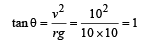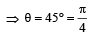31 Year NEET Previous Year Questions: Laws of Motion - 1 - Question 3

### Three blocks with masses m, 2 m and 3 m are connected by strings as shown in the figure. After an upward force, F is applied on block m, the masses move upward at constant speed v. What is the net force on the block of mass 2m? (g is the acceleration due to gravity)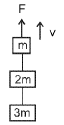Detailed Solution for 31 Year NEET Previous Year Questions: Laws of Motion - 1 - Question 3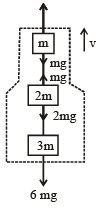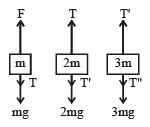From figure, F = 6 mg
As speed is constant, acceleration a = 0
∴ 6 mg = 6ma = 0
F = 6 mg
T = 5 mg , T' = 3 mg T" = 0
Fnet on block of mass 2 m = T – T' – 2 mg = (5 - 3 - 2)mg = 0
Alternate Method:
v  = constant  so, a = 0, Hence, Fnet = ma = 0

31 Year NEET Previous Year Questions: Laws of Motion - 1 - Question 4

A stone is dropped from a height of h. It hits the ground with a certain momentum P. If the same stone is dropped from a height 100% more than the previous height, the momentum when it hits the ground will change by:
[2012M]

Detailed Solution for 31 Year NEET Previous Year Questions: Laws of Motion - 1 - Question 4

Momentum,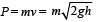(v2 = u2 + 2gh; Here u = 0)

When a stone hits the ground momentum: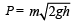When the same stone dropped from 2h (100% of initial) then momentum: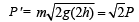which is changed by 41% of the initial.

or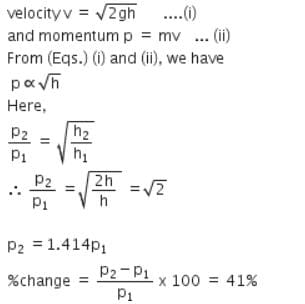31 Year NEET Previous Year Questions: Laws of Motion - 1 - Question 5

A car of mass 1000 kg negotiates a banked curve of a radius of 90 m on a frictionless road. If the banking angle is 45°, the speed of the car is:


Detailed Solution for 31 Year NEET Previous Year Questions: Laws of Motion - 1 - Question 5

For banking, tanθ = V2/ Rg
tan 45° = V/ 90*10 = 1
V = 30 m/s

31 Year NEET Previous Year Questions: Laws of Motion - 1 - Question 6

A conveyor belt is moving at a constant speed of 2m/s. A box is gently dropped on it. The coefficient of friction between them is µ = 0.5. The distance that the box will move relative to the belt before coming to rest on it taking g = 10 ms–2, is:


Detailed Solution for 31 Year NEET Previous Year Questions: Laws of Motion - 1 - Question 6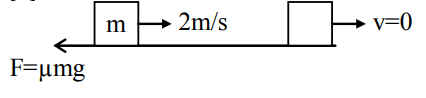Frictional force on the box, f = μmg
∴  Acceleration in the box, μg = 5 ms-2

v2 = u2 + 2as
0 = 22 + 2 * 5 * s
s = -2 / 5 w.r.t belt

Therefore distance = 0.4 m

31 Year NEET Previous Year Questions: Laws of Motion - 1 - Question 7

A body of mass M hits normally a rigid wall with velocity V and bounces back with the same velocity. The impulse experienced by the body is:


Detailed Solution for 31 Year NEET Previous Year Questions: Laws of Motion - 1 - Question 7

A Body of mass =m

velocity =V(i^)

Knock out (Bounce velocity) =V(−i^)

Change in momentum =m(v)−(m)(−v)
=2MV
The rate of change of momentum is Force
Thus dp/dt​​= force.
force × time = Impulse
Thus Impulse Experience by body =2mv.

31 Year NEET Previous Year Questions: Laws of Motion - 1 - Question 8

A person of mass 60 kg is inside a lift of mass 940 kg and presses the button on the control panel. The lift starts moving upwards with an acceleration 1.0 m/s2. If g = 10 ms–2, the tension in the supporting cable is:


Detailed Solution for 31 Year NEET Previous Year Questions: Laws of Motion - 1 - Question 8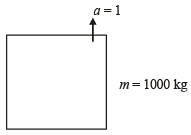Total mass = (60 + 940) kg = 1000 kg
The tension in the string is given as follows:

T=m(g+a)

By substituting the values of mass and acceleration given in the question we get

T=1000×(10+1)

=11000N

Hence, the tension in the string is11000N

31 Year NEET Previous Year Questions: Laws of Motion - 1 - Question 9

The mass of a lift is 2000 kg. When the tension in the supporting cable is 28000 N, then its acceleration is: 

Detailed Solution for 31 Year NEET Previous Year Questions: Laws of Motion - 1 - Question 9

The gravitational force in the downward direction is 20000 N and the tension force in the upward direction is 28000 N.

By newton's second law of motion, ΣF=ma

Net force, F = T – mg
ma = T – mg
2000 * a = 28000 – 20000 = 8000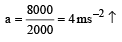31 Year NEET Previous Year Questions: Laws of Motion - 1 - Question 10

300 J of work is done in sliding a 2 kg block up an inclined plane of height 10 m. Taking g = 10 m/s2, work done against friction is:


Detailed Solution for 31 Year NEET Previous Year Questions: Laws of Motion - 1 - Question 10

Work done = 300J, Mass = 2kg, Height = 10m, g = 10m/s2
We know that the work done = f.d
300 = (mgsinθ + f) * (h / sinθ)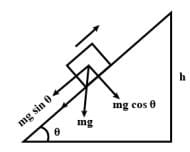Work done against the friction:
f.(h / sinθ) = 300 - mgsinθ * (h / sinθ)
300 - mgh = 300 - 2x10x10 = 100 J

31 Year NEET Previous Year Questions: Laws of Motion - 1 - Question 11

A block  B is pushed momen tarily along  a horizontal surface  with an initial velocity V. If μ is the coefficient of sliding friction  between B and the surface, block B will come to rest after a time ______.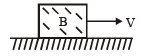Detailed Solution for 31 Year NEET Previous Year Questions: Laws of Motion - 1 - Question 11

Friction is the retarding force for the block F = ma = μR = μmg
From the first equation of motion:

v = u – at

0 = v−μgt

t = v​/μg

31 Year NEET Previous Year Questions: Laws of Motion - 1 - Question 12

A body under the action of a force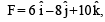acquires an acceleration of 1 m/s2. The mass of this body must be:

Detailed Solution for 31 Year NEET Previous Year Questions: Laws of Motion - 1 - Question 12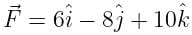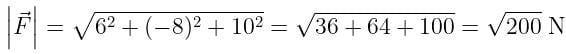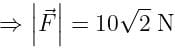Accelaration, a = 1 m/s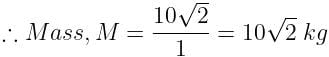31 Year NEET Previous Year Questions: Laws of Motion - 1 - Question 13

Sand is being dropped on a conveyor belt at the rate of M kg/s. The force necessary to keep the belt moving with a constant velocity of v m/s will be:

Detailed Solution for 31 Year NEET Previous Year Questions: Laws of Motion - 1 - Question 13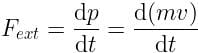m = mass of the system as a conveyor belt with sand drops at time t.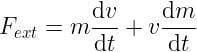∵ v → constant
∴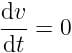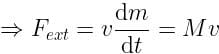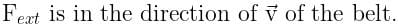31 Year NEET Previous Year Questions: Laws of Motion - 1 - Question 14

A block of mass m is placed on a smooth wedge of inclination θ. The whole system is accelerated horizontally so that the block does not slip on the wedge. The force exerted by the wedge on the block will be (g is acceleration due to gravity):

Detailed Solution for 31 Year NEET Previous Year Questions: Laws of Motion - 1 - Question 14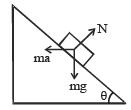According to Newton's second Law:

N = masinθ + mgcosθ     .....(1)
Also, mgsinθ = macosθ  .....(2)

From (1) & (2) we get, a = gtanθ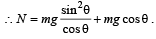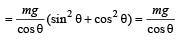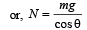31 Year NEET Previous Year Questions: Laws of Motion - 1 - Question 15

A car of mass m is moving on a level circular track of radius R. If μs represents the static friction between the road and tyres of the car, the maximum speed of the car in circular motion is given by:

Detailed Solution for 31 Year NEET Previous Year Questions: Laws of Motion - 1 - Question 15

For smooth driving maximum speed of car v then: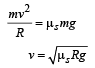## Physics Class 11

127 videos|464 docs|210 tests
 Use Code STAYHOME200 and get INR 200 additional OFF Use Coupon Code
Information about 31 Year NEET Previous Year Questions: Laws of Motion - 1 Page
In this test you can find the Exam questions for 31 Year NEET Previous Year Questions: Laws of Motion - 1 solved & explained in the simplest way possible. Besides giving Questions and answers for 31 Year NEET Previous Year Questions: Laws of Motion - 1, EduRev gives you an ample number of Online tests for practice

## Physics Class 11

127 videos|464 docs|210 tests

### How to Prepare for NEET

Read our guide to prepare for NEET which is created by Toppers & the best Teachers